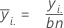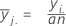# 均值分析中具有正态数据的双因子设计的方法和公式

## 均值

### 公式

 因子 A 的第 i 个水平的均值：因子 B 的第 j 个水平的均值：### 表示法

yi.因子 A 的第 i 个水平的所有观测值总和
y.j. 因子 B 的第 j 个水平的所有观测值的总和
aA 中的水平数
bB 中的水平数
n分别位于因子 A 的第 i 个水平和因子 B 的第 j 个水平的案例数

## 总平均值（中心线）

### 表示法

y...样本中所有观测值的总和
aA 中的水平数
bB 中的水平数
n分别位于因子 A 的第 i 个水平和因子 B 的第 j 个水平的案例数

## 主效应的决策上限和下限

• UDLA =...+ (.5) * hα* Sqrt[(MSE) * (2 / n1)]
• LDLA =...- (.5) * hα* Sqrt[(MSE) * (2 / n1)]

• UDLA =...+ hα* Sqrt[MSE * (a - 1) / (a * n1)]
• LDLA =...- hα* Sqrt[MSE * (a - 1) / (a * n1)]

• UDLA =...+ hα* Sqrt[MSE * (a - 1) / (a * n1)]
• LDLA =...- hα* Sqrt[MSE * (a - 1) / (a * n1)]

where MSE = mean square error (from an ANOVA with terms A, B, and AB), n1= number of observations at each level of the factor. and hα = absolute value (t(α2, df); where a2 = (1- (1- a )** (1 / a)) / 2 and df = nT - ab where nT = total number of observations in the sample,.

1. L.S.Nelson (1983)。“Exact Critical Values for Use with the Analysis of Means”（用于均值分析的精确临界值），Journal of Quality Technology（质量技术期刊），第 15 期，第 40-44 页。

## 交互效应的决策上限和下限

• UDLA B = hα* Sqrt[MSE * (q / (a * b * n)]
• LDLA B = –hα* Sqrt[MSE * (q / (a * b * n)]

• α2 = a / 2

• α2 = (1- (1- a )** (1 / b)) / 2

• α2 = (1- (1- a )** (1 / a)) / 2

• α2 = (1- (1- a )** (1 /a * b)) / 2